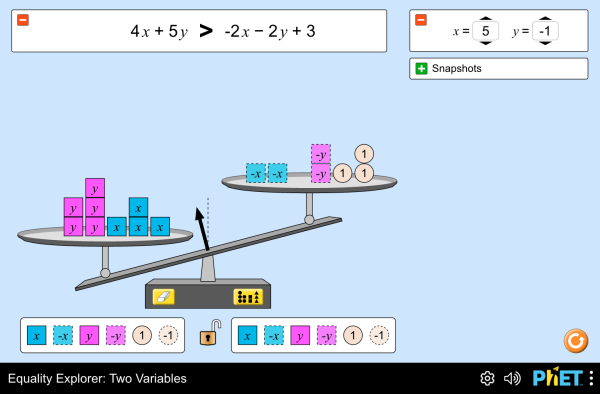# 等式探索:两个变量下载嵌入代码 关闭 嵌入模拟程序控制 你可以通过改变嵌入的代码改变程序的宽度和高度 插入一张图片，当点击这个图片时启动仿真程序。

### 主题

• Equations
• Inequalities
• System of Equations

### 示范 学习目标

• Solve and manipulate algebraic equations by substituting different values for a variable
• Use a balance model to solve an equation for an unknown, and justify your strategies for solving
• Build a system of equations
• Determine the solution set of a system of equations

### 标准对齐

#### Common Core - Math

6.EE.B.5
Understand solving an equation or inequality as a process of answering a question: which values from a specified set, if any, make the equation or inequality true? Use substitution to determine whether a given number in a specified set makes an equation or inequality true.
6.EE.B.6
Use variables to represent numbers and write expressions when solving a real-world or mathematical problem; understand that a variable can represent an unknown number, or, depending on the purpose at hand, any number in a specified set.
7.EE.B.3
Solve multi-step real-life and mathematical problems posed with positive and negative rational numbers in any form (whole numbers, fractions, and decimals), using tools strategically. Apply properties of operations to calculate with numbers in any form; convert between forms as appropriate; and assess the reasonableness of answers using mental computation and estimation strategies. For example: If a woman making \$25 an hour gets a 10% raise, she will make an additional 1/10 of her salary an hour, or \$2.50, for a new salary of \$27.50. If you want to place a towel bar 9 3/4 inches long in the center of a door that is 27 1/2 inches wide, you will need to place the bar about 9 inches from each edge; this estimate can be used as a check on the exact computation.
7.EE.B.4
Use variables to represent quantities in a real-world or mathematical problem, and construct simple equations and inequalities to solve problems by reasoning about the quantities.
8.EE.C.7
Solve linear equations in one variable.
8.EE.C.7a
Give examples of linear equations in one variable with one solution, infinitely many solutions, or no solutions. Show which of these possibilities is the case by successively transforming the given equation into simpler forms, until an equivalent equation of the form x = a, a = a, or a = b results (where a and b are different numbers).
8.EE.C.8
Analyze and solve pairs of simultaneous linear equations.
HSA-CED.A.1
Create equations and inequalities in one variable and use them to solve problems. Include equations arising from linear and quadratic functions, and simple rational and exponential functions.
HSA-CED.A.3
Represent constraints by equations or inequalities, and by systems of equations and/or inequalities, and interpret solutions as viable or nonviable options in a modeling context. For example, represent inequalities describing nutritional and cost constraints on combinations of different foods.
HSA-REI.A.1
Explain each step in solving a simple equation as following from the equality of numbers asserted at the previous step, starting from the assumption that the original equation has a solution. Construct a viable argument to justify a solution method.
HSA-REI.B.3
Solve linear equations and inequalities in one variable, including equations with coefficients represented by letters.

### 教师指南仿真程序控制概述,简化模型,学生视角的思考 ( PDF ).

### 教师提交的活动

SECUNDARIA: Alineación PhET con programas de la SEP México (2011 y 2017)Diana López 初中

PREPARATORIA: Alineación de PhET con programas de la DGB México (2017)Diana López 高中

Middle School Math Sim AlignmentAmanda McGarry 初中 其它 数学

### HTML5 程序：

iOS 8+ Safari

Android:

Chromebook:

Windows 系统:

Macintosh 系统:
OS 10.9.5 或之后的系统版本，Safari 9+版本，或者火狐浏览器，谷歌浏览器的最新版本均支持我们的程序。

Linux 系统:

### Java and Flash 程序：

Java 与 Flash程序可以在PC端，mac端，及linux系统上运行。具体的系统配置要求如下：

Windows 系统:
Windows 10, 8.1, 7

Macintosh 系统:
Mac OS X 10.9.5+

64位浏览器（例如Safari，火狐，谷歌等）

Linux 系统:
Oracle Linux 5.5+1
Oracle Linux 6.x (32位), 6.x (64位)2
Oracle Linux 7.x (64位)2
Red Hat Enterprise Linux 5.5+1, 6.x (32位), 6.x (64位)2
Ubuntu Linux 12.04 LTS, 13.x
Suse Linux Enterprise Server 10 SP2+, 11.x

• Chris Malley (developer)
• Diana López Tavares
• Ariel Paul
• Kathy Perkins
• Argenta Price
• David Webb
• Mariah Hermsmeyer (graphic arts)
• Cheryl McCutchan (graphic arts)
• almond-0.2.9.js
• base64-js-1.2.0.js
• easing-equations-r12
• FileSaver-b8054a2.js
• font-awesome-4.5.0
• game-up-camera-1.0.0.js
• he-1.1.1.js
• himalaya-0.2.7.js
• jama-1.0.2
• jquery-2.1.0.js
• lodash-4.17.4.js
• pegjs-0.7.0.js
• seedrandom-2.4.2.js
• text-2.0.12.js
• TextEncoderLite-3c9f6f0.js
• Tween-r12.js